Ex 9.2

Chapter 9 Class 11 Sequences and Series
Serial order wise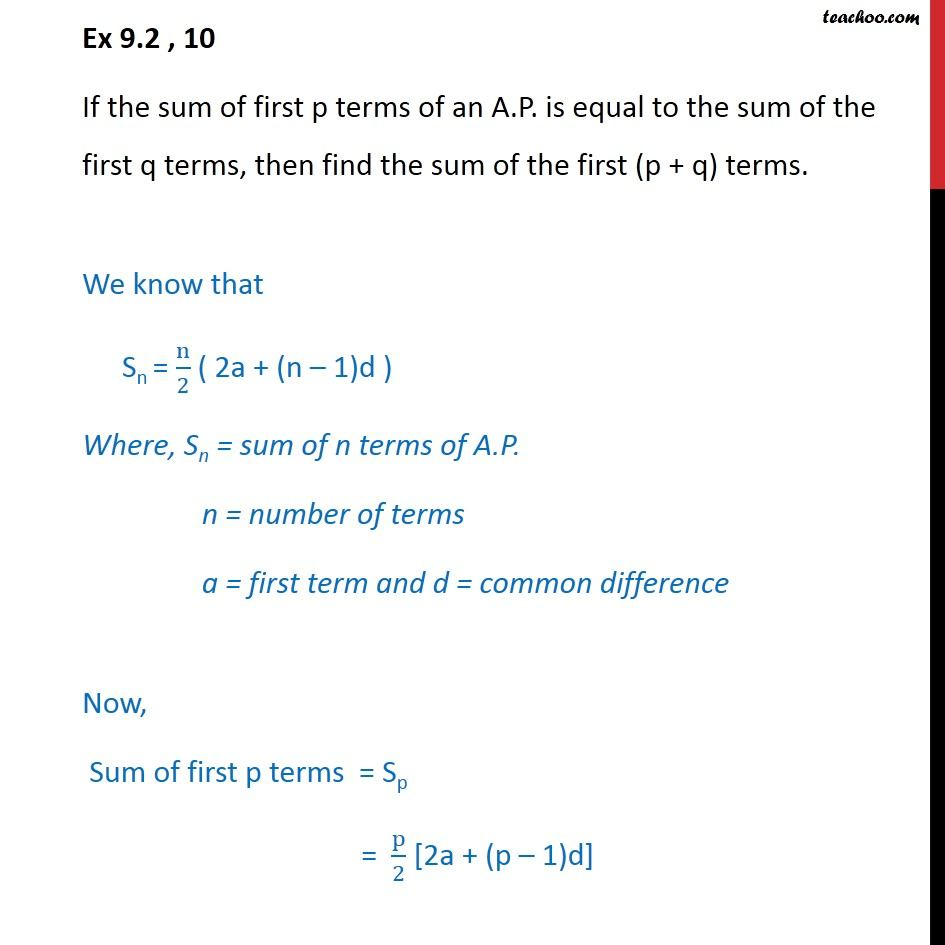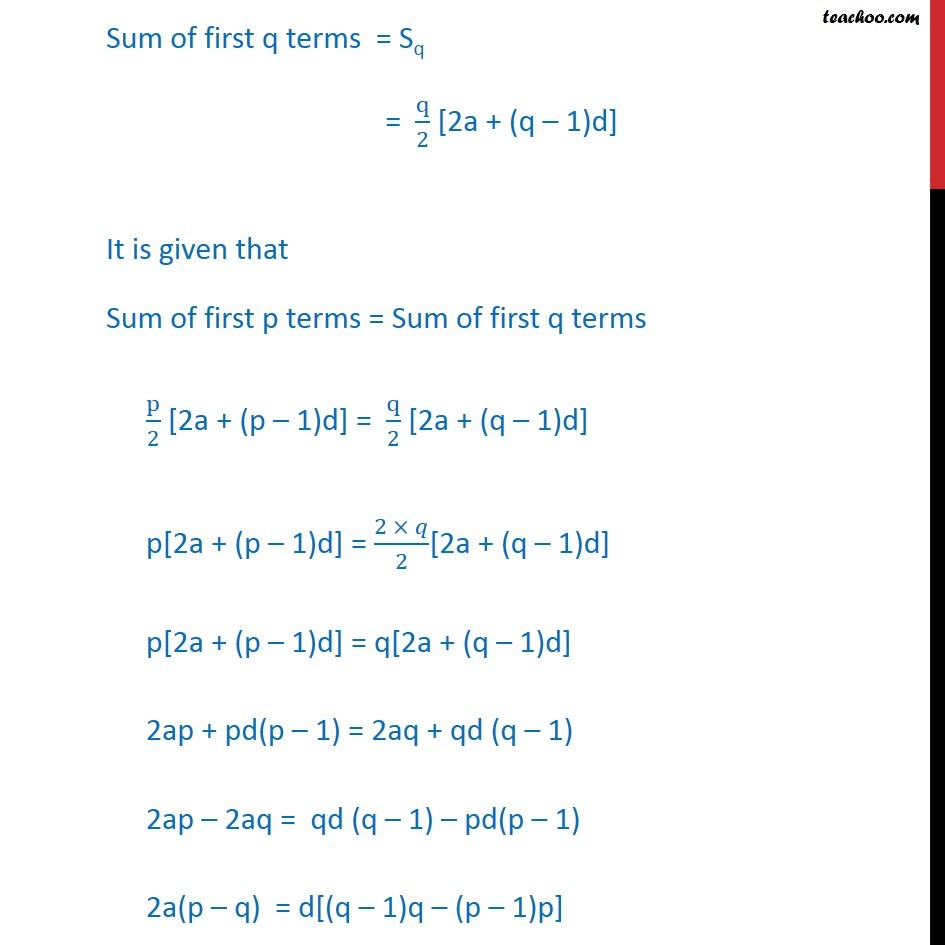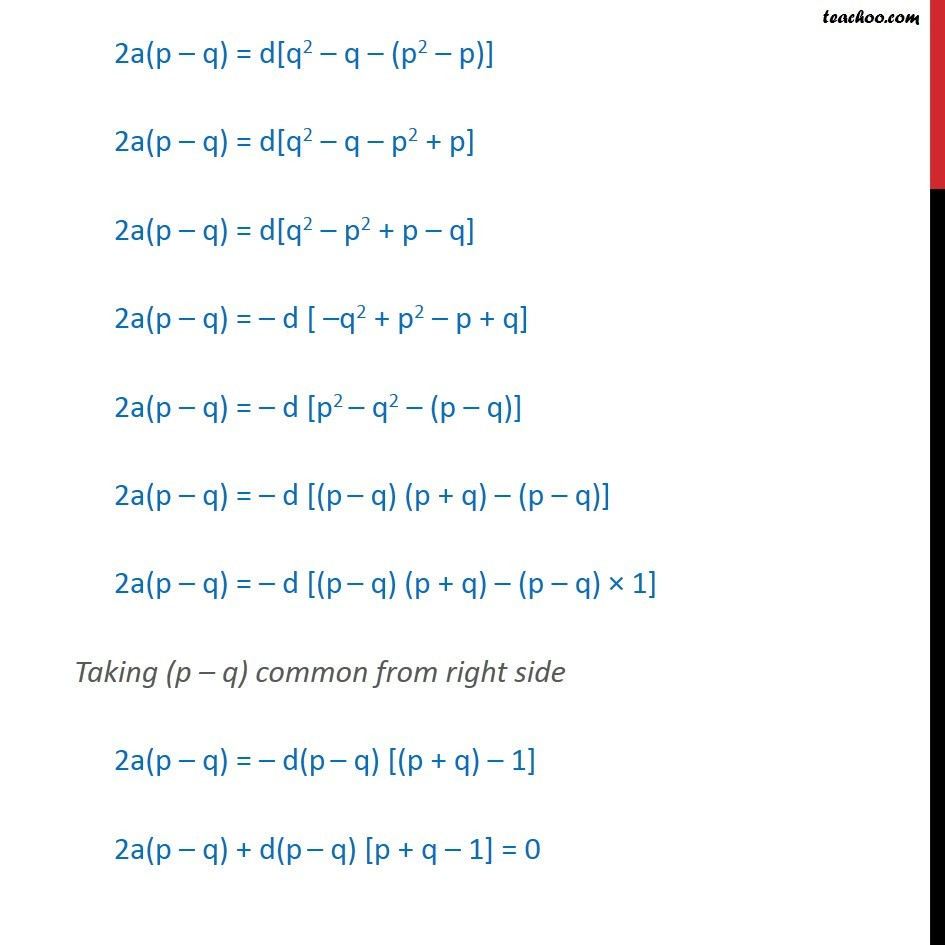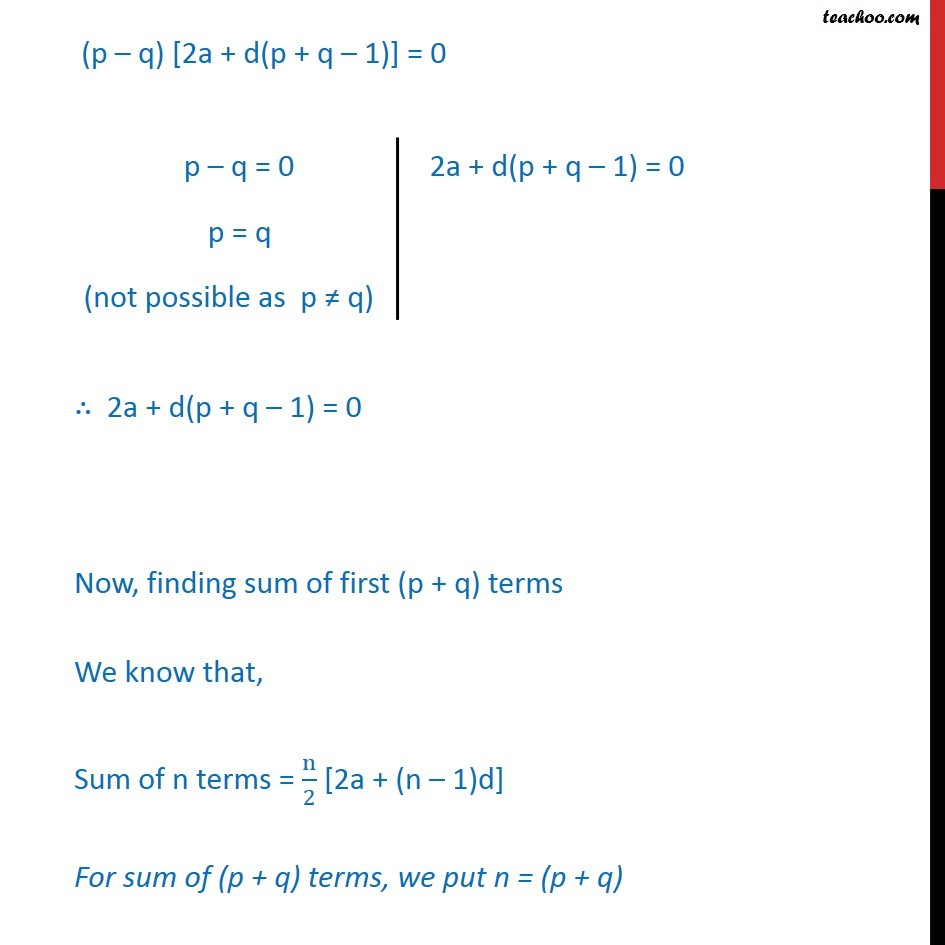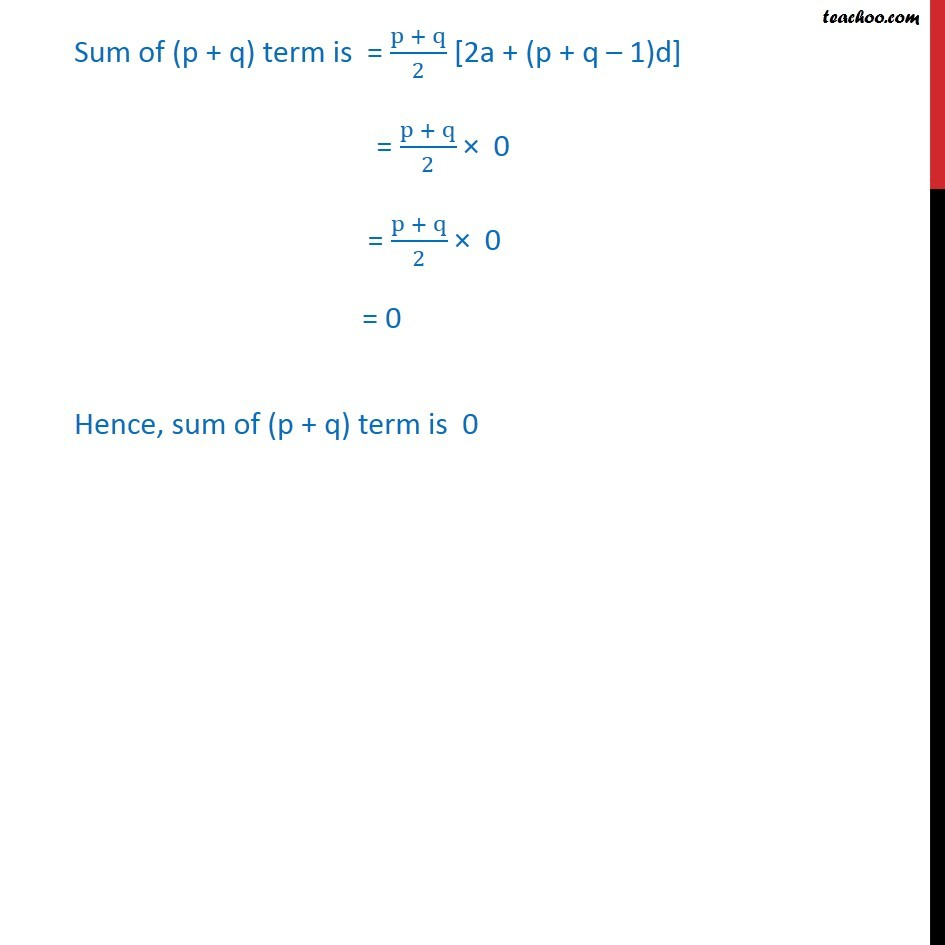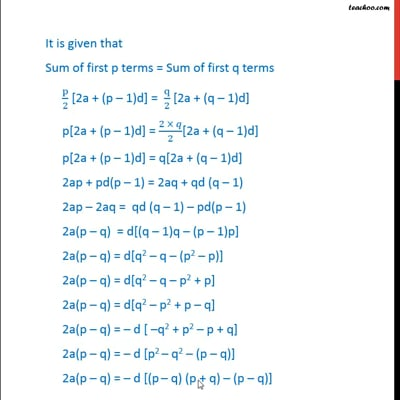This video is only available for Teachoo black users

Solve all your doubts with Teachoo Black (new monthly pack available now!)

### Transcript

Ex9.2 , 10 If the sum of first p terms of an A.P. is equal to the sum of the first q terms, then find the sum of the first (p + q) terms. We know that Sn = n/2 ( 2a + (n 1)d ) Where, Sn = sum of n terms of A.P. n = number of terms a = first term and d = common difference Now, Sum of first p terms = Sp = p/2 [2a + (p 1)d] Sum of first q terms = Sq = q/2 [2a + (q 1)d] It is given that Sum of first p terms = Sum of first q terms p/2 [2a + (p 1)d] = q/2 [2a + (q 1)d] p[2a + (p 1)d] = (2 )/2[2a + (q 1)d] p[2a + (p 1)d] = q[2a + (q 1)d] 2ap + pd(p 1) = 2aq + qd (q 1) 2ap 2aq = qd (q 1) pd(p 1) 2a(p q) = d[(q 1)q (p 1)p] 2a(p q) = d[q2 q (p2 p)] 2a(p q) = d[q2 q p2 + p] 2a(p q) = d[q2 p2 + p q] 2a(p q) = d [ q2 + p2 p + q] 2a(p q) = d [p2 q2 (p q)] 2a(p q) = d [(p q) (p + q) (p q)] 2a(p q) = d(p q) [p + q 1] 2a(p q) + d(p q) [p + q 1] = 0 (p q) [2a + d(p + q 1)] = 0 2a + d(p + q 1) = 0 Now, finding sum of first (p + q) terms We know that, Sum of n terms = n/2 [2a + (n 1)d] For sum of (p + q) terms, we put n = (p + q) Sum of (p + q) term is = (p + q)/2 [2a + (p + q 1)d] = (p + q)/2 0 = 0 Hence, sum of (p + q) term is 0